# Digital Electronics - Combinational Logic Analysis

### Exercise :: Combinational Logic Analysis - General Questions

21.

Implementation of the Boolean expression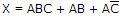results in ________.

 A. three AND gates, one OR gate B. three AND gates, one NOT gate, one OR gate C. three AND gates, one NOT gate, three OR gates D. three AND gates, three OR gates

Answer: Option B

Explanation:

No answer description available for this question. Let us discuss.

22.

One possible output expression for an AND-OR-Invert circuit having one AND gate with inputs A, B, and C and one AND gate with inputs D and E is ________.

 A.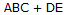B.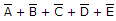C.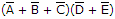D.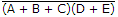Answer: Option C

Explanation:

No answer description available for this question. Let us discuss.

23.

How many 2-input NOR gates does it take to produce a 2-input NAND gate?

 A. 1 B. 2 C. 3 D. 4

Answer: Option D

Explanation:

No answer description available for this question. Let us discuss.

24.

A logic circuit with an output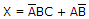consists of ________.

 A. two AND gates, two OR gates, two inverters B. three AND gates, two OR gates, one inverter C. two AND gates, one OR gate, two inverters D. two AND gates, one OR gate

Answer: Option C

Explanation:

No answer description available for this question. Let us discuss.

#### Current Affairs 2021

Interview Questions and Answers